## Home for HMNL Games and Personal Software

### Recent News

•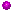Just Released
•Coming Soon
•Welcome

# Games and Personal Software

#### A Bit of CS

##### Bruce Incognito  21 July 2013 00:17:49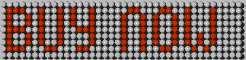## A BIT OF COMPUTER SCIENCE

### Introduction

The "eight queens" problem was first proposed by the German chess player Max Bezzel in 1848. Many mathematicians dabbled with the problem including, most notably, Carl Friedrich Gauss. The first solution to the problem was given in 1850 by Franz Nauck, who then followed this with a solution to the generalised n-Queens problem (on an n x n chessboard) in 1874. Many mathematicians and computer scientists have since studied the problem or used it to illustrate theorem proving or problem solving techniques. Perhaps most famously the late, great Edsger Dijkstra used the problem in 1972 to illustrate the power of "Structured Programming". Most recently the problem is used in the teaching of aspects of logic programming languages such as Prolog, in this scenario the problem is often posed as "find the number of solutions to the eight queens (or the more generalised n-Queens) problem".

### How Many Solutions Are There to the Eight Queens Problem?

In the style of Douglas Adams I could just leave you with the ultimate answer - 92.
However I suspect that as you have read this far you probably expect a little more explanation and information.
In the discussion below queens are described by their position on the chessboard expressed as a Row co-ordinate (1 - 8) and a Column co-ordinate (1 - 8), all aspects of the discussion also pertain to the more generalised n-Queens problem.
We will start by looking at a program to answer the question about how many solutions there are.

#### Stating the Problem

Find all possible arrangements of 8 queens placed on an 8 x 8 chessboard such that the arrangement satisfies the three following constraints.

 1. No two queens reside on the same row of the board. 2. No two queens reside on the same column of the board. 3. No two queens reside on the same diagonal of the board

We now need to decide how we will represent the problem programmatically so that we can answer the question, we have to create an abstraction of the real-world problem in such a way that we can derive the answer through computation. This part of the process is often skipped over and neglected in many Computer Science courses and should not be, as this is the most creative and critical step. A sage word of advice here is to never neglect the obvious, just because some aspect is obvious does not mean that it is either trivial or irrelevant.
It is obvious (but important) from the first constraint that there must be exactly one queen on each row of the board and it is equally obvious (but equally important) that there must be exactly one queen on each column of the board.

It is a very small conceptual step to see from these obvious observations that we can encode the state of the board containing the 8 queens as a simple one-dimensional array with 8 cells each representing the different rows on the board and each cell containing a unique integer from 1 to 8 representing the column containing the queen on the row given by the index in the array.

This seems to be a nice compact method of encoding an arrangement of the board but further we observe that the encoding that we have selected actually removes constraints 1 and 2 from any further consideration as they are always satisfied by any arrangement encoded in this manner, cute eh! This compact form for encoding the problem was first proposed by Gauss in correspondence with his friend the astronomer H.C. Schumacher in 1850.

Further we observe that if we populate an initial arrangement of the array as representing the queens on a single diagonal from top left to bottom right i.e. Array = [1,2,3,4,5,6,7,8], we can now see that the abstract problem can be stated as:

Find every permutation of the array [1,2,3,4,5,6,7,8] that satisfies constraint 3.

So how do we test an arrangement to make sure that it satisfies constraint 3? Any two queens can be tested to ensure that they are not on the same diagonal by testing that the absolute (ignore the sign) of the difference of their respective rows is not equal to the absolute difference of their columns. We can therefore ensure that a complete arrangement satisfies constraint 3 by testing each queen against each of the other queens to ensure that they all satisfy the inequality.

#### A Bit More Formally

Find every permutation of the array [1,2,3,4,5,6,7,8] where no pairs of elements (e1, e2) of the array satisfy the condition abs(R(e1) - R(e2)) == abs(e1 - e2). Where R(e1) is the 1 based index of the element e1 in the array.

#### Solving the Problem in 'C'

Let us start off by solving the problem in a functional language (let's pick 'C' as an example. The code presented is meant to be illustrative and clear, not stylish!
We will present this "bottom up" starting with a function for testing that an arrangement satisfies constraint 3.

 //  Test arrangementint testArrangement(int FArray[]){    int                                iIndex,jIndex;                  //  Generic inexes    for (iIndex = 0; iIndex 7; iIndex++)    {        for (jIndex = iIndex + 1; jIndex 8; jIndex++)        {            //  Test for a collision on a diagonal            if ((jIndex - iIndex) =abs(FArray[jIndex] - FArray[iIndex])) return 0;        }    }    printf("INFO: Solution found: [%i,%i,%i,%i,%i,%i,%i,%i].\r\n",    FArray,    FArray,    FArray,    FArray,    FArray,    FArray,    FArray,    FArray);    return 1;                               //  Show that another solution was found}

There is nothing too startling about the code, as the inequality is commutative we are able to optimise the two loops a bit by not performing any tests that we have already done the other way round i.e we don't test row 5 against row 1 because we have already tested row 1 against row 5 and so on.

All that we have to do now is to generate every permutation of the array and pass them in turn to the function to test each arrangement and count up the number of arrangements that satisfy constraint 3. We will use a standard divide and conquer recursive generator to produce the permutations of the array that contains the arrangement.

It should be noted that it is perfectly possible to traverse the complete solution graph recursively, however, in this implementation we sequentially process the breadth of the graph at each level.

 //  Permute Arrayint permuteArray(int FArray[], int FAC, int VArray[], int VAC){    int                        SolutionsFound = 0;                 //  Count of solutions found    int                        NewFArray = {0,0,0,0,0,0,0,0};   //  New fixed array    int                        NewVArray = {0,0,0,0,0,0,0,0};   //  New variable array    int                        iIndex,jIndex,kIndex;               //  Generic indexes    //  Copy the fixed and variable arrays    for (iIndex = 0; iIndex 8; iIndex++)    {        NewFArray[iIndex] = FArray[iIndex];    }    //  Test for the boundary condition - There is only a single element in the variable array    if (VAC == 1)    {        //  Complete the permutation        NewFArray = VArray;        SolutionsFound = SolutionsFound + testArrangement(NewFArray);        return SolutionsFound;    }    //  Move one element from the variable array to the fixed array and recursively    //  invoke the permuter    for (iIndex = VAC - 1; iIndex >= 0; iIndex--)    {        NewFArray[FAC] = VArray[iIndex];        kIndex = 0;        for (jIndex = 0; jIndex VAC; jIndex++)        {            if (VArray[jIndex] != NewFArray[FAC])            {                NewVArray[kIndex] = VArray[jIndex];                kIndex++;            }        }        SolutionsFound SolutionsFound + permuteArray(NewFArray, FAC + 1, NewVArray, VAC - 1);    }    return SolutionsFound;}

The function takes each element in the variable array (VArray) and moves it to the next available position in the fixed array (FArray) and then recursively calls the permutation function to do the same again until there is only one element remaining in the variable array. At that point it adds the last element to the fixed array and then tests that arrangement for for compliance with constraint 3 by calling the testArrangement() function. The function returns the number of satisfying arrangements found in the branch of the permutation tree that it was called with.

All that remains now is to call the permutation generator with the initial states of the arrays i.e. the fixed array empty and the variable array containing all elements and then report on the number of satisfying solutions found.

 //  Main Entry Pointint main(int argc, char *argv[]){    int                        EArray = {0,0,0,0,0,0,0,0};                //  Empty array    int                        IArray = {1,2,3,4,5,6,7,8};                //  Initial array    int                        Solutions;                                    //  Count of solutions    //  Invoke the permuter to generate the permutations    Solutions = permuteArray(EArray, 0, IArray, 8);    //  Show how many solutions were found    printf("INFO: Run completed %i solutions discovered.\r\n", Solutions);    return 0;}

All that remains is to add a little preamble and the complete application can be compiled, linked and run.

 //  Language Includes#include       #include       //  Function prototypes/Forward declarationsint permuteArray(int FArray[], int FAC, int VArray[], int VAC);int testArrangement(int FArray[]);

Running the program yields a list of each satisfying arrangement found along with the total count.

 ........INFO: Solution found: [2,5,7,1,3,8,6,4].INFO: Solution found: [2,4,6,8,3,1,7,5].INFO: Solution found: [1,7,5,8,2,4,6,3].INFO: Solution found: [1,7,4,6,8,2,5,3].INFO: Solution found: [1,6,8,3,7,4,2,5].INFO: Solution found: [1,5,8,6,3,7,2,4].INFO: Run completed 92 solutions discovered.

The simplicity and elegance of the program to list and count the satisfying arrangements is all down to that important first step of problem abstraction, the importance of which cannot be overemphasised. As an interesting comparison we will solve the same problem in a declarative language such as Prolog.

#### Solving the Problem in Prolog

We will use exactly the same problem abstraction to solve the problem in Prolog that we used for the 'C' program. Again we will work bottom up starting with the predicates that are used to test that an arrangement satisfies the constraints.

 %%  The following predicates test that the current board arrangement satisfies the constraints%testArrangement([_]) :- !.testArrangement([TheQueen | OtherQueens]) :- !, notake(TheQueen, 1, OtherQueens), testArrangement(OtherQueens).notake(_, _, []) :- !.notake(TheQueen, DeltaRow, [NextQueen|OtherQueens]) :- !,                 Hit1 is TheQueen + DeltaRow,                 NextQueen =\= Hit1,                 Hit2 is TheQueen - DeltaRow,                 NextQueen =\= Hit2,                 NewDeltaRow is DeltaRow + 1,                 notake(TheQueen, NewDeltaRow, OtherQueens).

Because we will be using backtracking to find all of the possible solutions we will need a couple of additional predicates to enable us to keep track of the count of solutions found.

 %%  The following predicates count the solutions%initialiseCounter(I) :- retractall(counter(_)), assert(counter(I)).incrementCounter(I) :- retract(counter(C)), N is C + I, assert(counter(N)), !.

We now add predicates to generate each of the possible arrangements and test each to see if they satisfy the constraints.

 %%  The following predicates generates a permutation of the arrangement%listInsert(A, B, [A | B]).listInsert(A, [B1 | B2], [B1 | C]) :- listInsert(A, B2, C).permuteArray([A], [A]).permuteArray([A | B], C) :- permuteArray(B, C1), listInsert(A, C1, C).%%  The following predicates find all of the solutions%findSolution(A, B) :- permuteArray(A, B), testArrangement(B).findAllSolutions(A) :- initialiseCounter(0), findSolution(A, B), incrementCounter(1), counter(X), print(X), print('. '), print(B), nl, fail.findAllSolutions(_) :- nl,print('INFO: Run completed '), counter(X), print(X), print(' solutions found.'),nl.

All we need to do now is to trigger the search for all of the solutions by querying the prolog database with the initial arrangement.

 ?- findAllSolutions([1,2,3,4,5,6,7,8]).........87. [4,8,5,3,1,7,2,6]88. [4,2,8,5,7,1,3,6]89. [4,8,1,5,7,2,6,3]90. [8,2,5,3,1,7,4,6]91. [8,2,4,1,7,5,3,6]92. [3,8,4,7,1,6,2,5]INFO: Run completed 92 solutions found.

All well and good, we have convinced ourselves that there are 92 solutions to the eight queens problem but we know nothing more than that. We have no clues as to why there should be 92 solutions or how these solutions are formed.

### Some Geometry of the Eight Queens Problem

We start off by taking a board arrangement that we discovered in the brute force searches, the compact encoding for this arrangement is [3,8,4,7,1,6,2,5] which was solution 92 from the prolog run to find all solutions.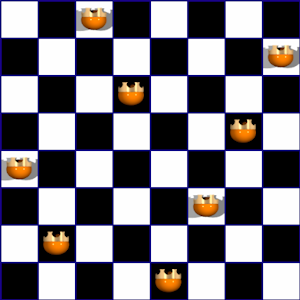We observe that we can rotate the board through 90 degrees clockwise and we have generated another valid arrangement with the compact encoding [4,2,8,6,1,3,5,7]. This makes sense as we would expect all three constraints for a valid arrangement to hold true under rotation. A quick look at the transformation function for a 90 degree clockwise rotation (R, C) -> (C, 9 - R)
and the inequalities in our constraints will show that the 90 degree clockwise rotation will always result in another satisfying arrangement.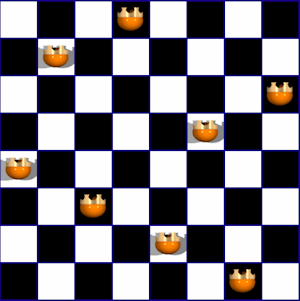As a single clockwise rotation by 90 degrees yields another unique satisfying arrangement then it should be clear that we can rotate the new arrangement by 90 degrees clockwise again to give yet another satisfying arrangement and the same yet again. These additional rotations give us [4,7,3,8,2,5,1,6] and [2,4,6,8,3,1,7,5].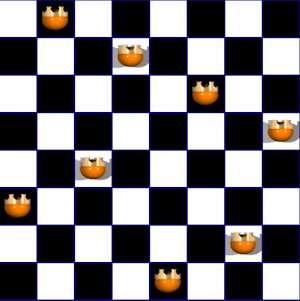We further observe that if we reflect a satisfying arrangement about the bottom edge of the board we derive yet another satisfying arrangement [5,2,6,1,7,4,8,3]. Again a quick look at the transformation function for the reflection (R, C) -> (9 - R, C) and the inequalities in the constraints shows that again this transform will also yield a satisfying arrangement.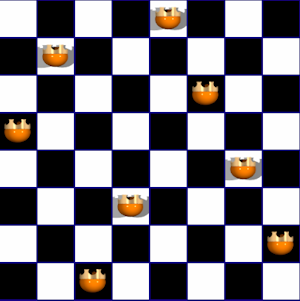Of course we can then apply the 90 degree clockwise rotation transform to the result of the reflection to give us another three satisfying arrangements [5,7,1,3,8,6,4,2], [6,1,5,2,8,3,7,4] and [7,5,3,1,6,8,2,4].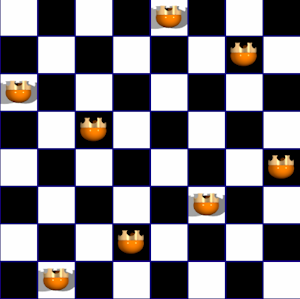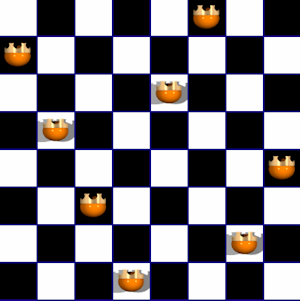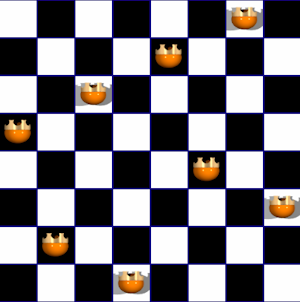So we have made some significant progress in finding some underlying structure to the set of satisfying arrangements for the eight queens problem. We would expect the full set of solution to come in sets of 8 arrangements that represent the untransformed arrangement plus the seven possible transforms identified above. But hold on a moment our brute force search identified a total of 92 satisfying arrangements and that is not a multiple of 8!

Some analysis of the results from the brute force search reveals that there are in fact 12 unique groups of satisfying arrangements, 11 of which have 8 distinct arrangements in the set and the one remaining sent only yields 4 distinct satisfying arrangements.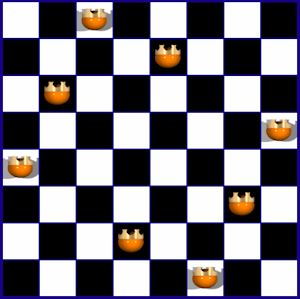Untransformed [3,5,2,8,1,7,4,6]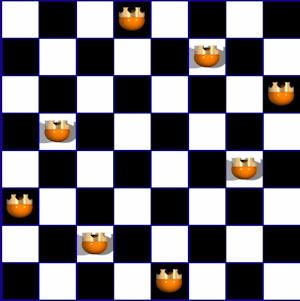Rotate 90 [4,6,8,2,7,1,3,5]Rotate 180 [3,5,2,8,1,7,4,6]Rotate 270 [4,6,8,2,7,1,3,5]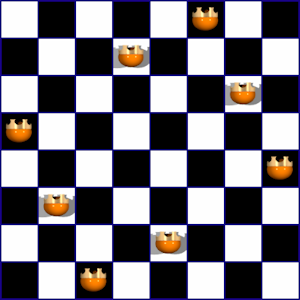Reflect [6,4,7,1,8,2,5,3]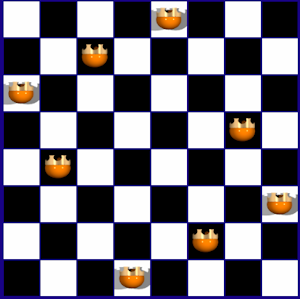Reflect + Rotate 90 [5,3,1,7,2,8,6,4]Reflect + Rotate 180 [6,4,7,1,8,2,5,3]Reflect + Rotate 270 [5,3,1,7,2,8,6,4]

The reason that this particular set of arrangements produces isomorphic forms should be readily apparent. The arrangement has two axes of internal rotational symmetry. Split any one of the arrangements in the set in half about the horizontal or the vertical axis and then rotate one of the halves by 180 degrees and the symmetry is readily apparent.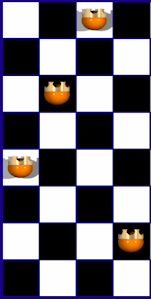Left Hand Side of [3,5,2,8,1,7,4,6]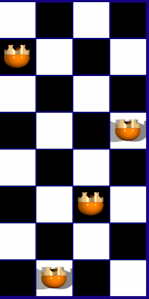Right Hand Side of [3,5,2,8,1,7,4,6]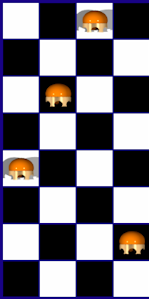Right Hand Side Rotated 180 degrees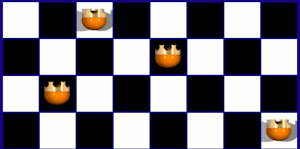Top Half of [3,5,2,8,1,7,4,6]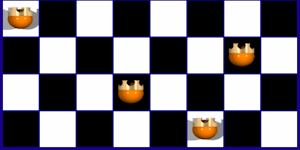Bottom Half of [3,5,2,8,1,7,4,6]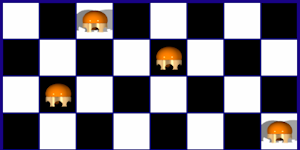Bottom Half Rotated 180 degrees

Enough of the structure of the solutions to the "eight queens" problem, click on the following link to find out more about how we use Computer Science in the Q8 Game.

### Q8

•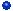About Q8
•Playing Q8
•Q8 Opponents
•A Bit of Computer Science
•Computer Science in Q8
•Big Pink Buttons
•Q8 FAQ
•Squiffy FAQ
•Problems with Q8